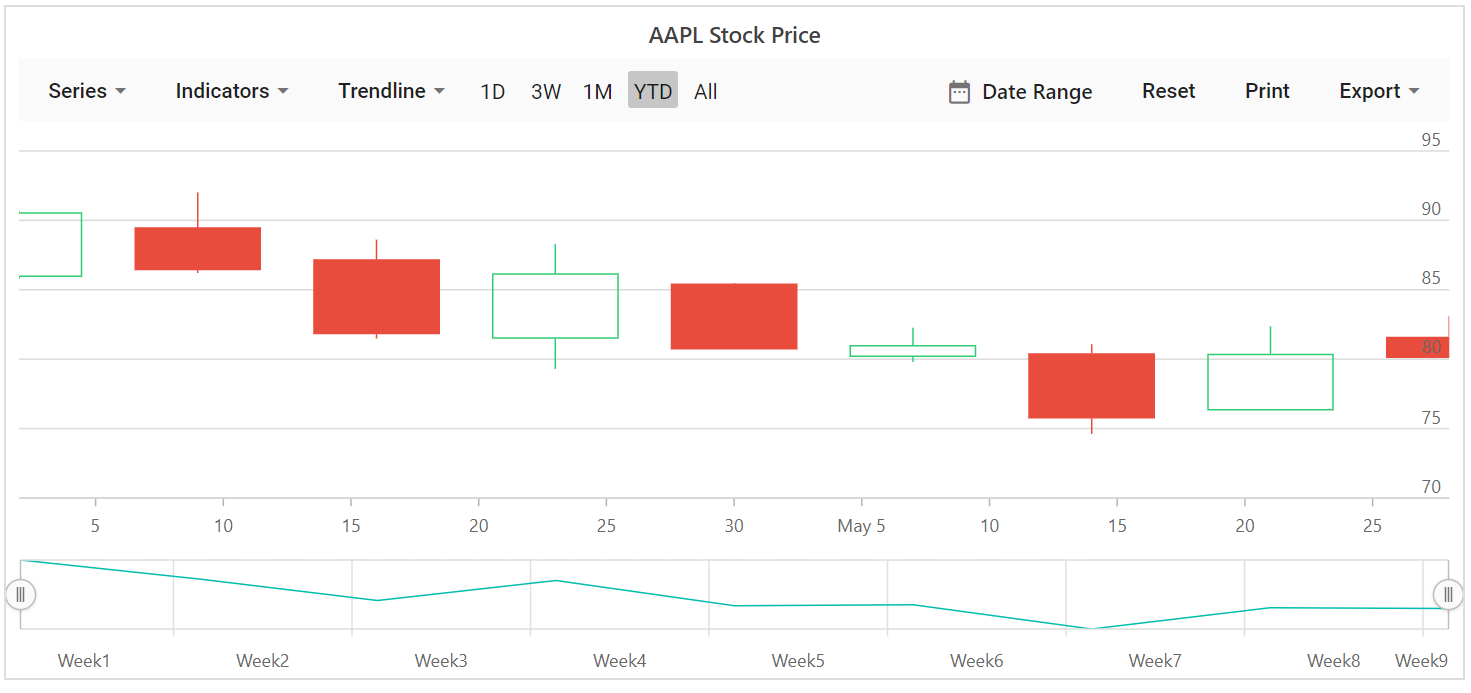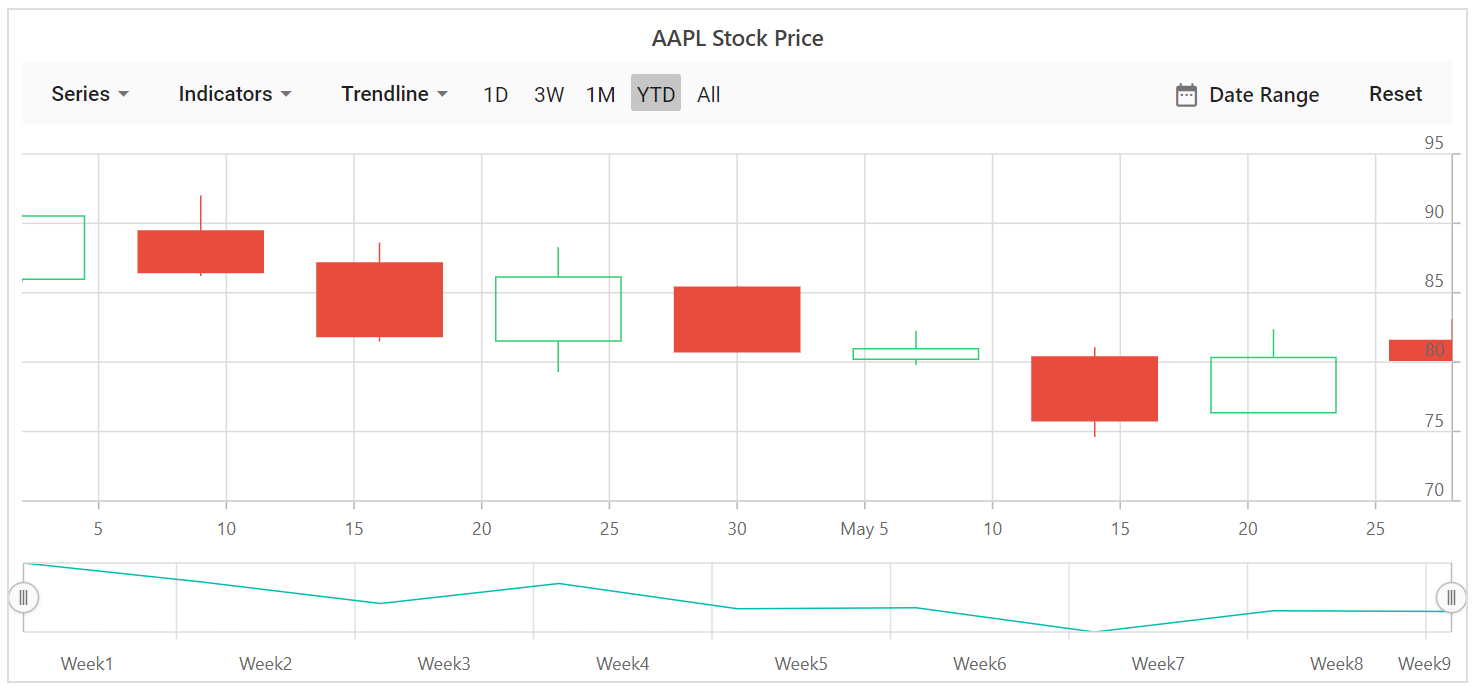# Print and Export in Blazor Stock Chart Component

30 Nov 20224 minutes to read

The rendered stock chart can be exported to `JPEG`, `PNG`, `SVG`, or `PDF` format using the export dropdown button in the period selector toolbar. The required format can be chosen using the export dropdown button in stock-chart.

The rendered stock chart can be printed directly using print button in period selector toolbar.

``````@using Syncfusion.Blazor.Charts

<SfStockChart Title="AAPL Stock Price">
<StockChartSeriesCollection>
<StockChartSeries DataSource="@StockDetails" Type="ChartSeriesType.Candle" XName="Date" High="High" Low="Low" Open="Open" Close="Close" Volume="Volume"></StockChartSeries>
</StockChartSeriesCollection>
<StockChartPrimaryYAxis>
<StockChartAxisLineStyle Color="Transparent"></StockChartAxisLineStyle>
<StockChartAxisMajorTickLines Color="Transparent" Width="0"></StockChartAxisMajorTickLines>
</StockChartPrimaryYAxis>
<StockChartPrimaryXAxis>
<StockChartAxisMajorGridLines Color="Transparent"></StockChartAxisMajorGridLines>
</StockChartPrimaryXAxis>
</SfStockChart>

@code {

public class ChartData
{
public DateTime Date {get; set;}
public Double Open {get; set;}
public Double Low {get; set;}
public Double Close {get; set;}
public Double High {get; set;}
public Double Volume {get; set;}
}

public List<ChartData> StockDetails = new List<ChartData>
{
new ChartData { Date = new DateTime(2012, 04, 02), Open= 85.9757, High = 90.6657,Low = 85.7685, Close = 90.5257,Volume = 660187068},
new ChartData { Date = new DateTime(2012, 04, 09), Open= 89.4471, High = 92,Low = 86.2157, Close = 86.4614,Volume = 912634864},
new ChartData { Date = new DateTime(2012, 04, 16), Open= 87.1514, High = 88.6071,Low = 81.4885, Close = 81.8543,Volume = 1221746066},
new ChartData { Date = new DateTime(2012, 04, 23), Open= 81.5157, High = 88.2857,Low = 79.2857, Close = 86.1428,Volume = 965935749},
new ChartData { Date = new DateTime(2012, 04, 30), Open= 85.4, High =  85.4857,Low = 80.7385, Close = 80.75,Volume = 615249365},
new ChartData { Date = new DateTime(2012, 05, 07), Open= 80.2143, High = 82.2685,Low = 79.8185, Close = 80.9585,Volume = 541742692},
new ChartData { Date = new DateTime(2012, 05, 14), Open= 80.3671, High = 81.0728,Low = 74.5971, Close = 75.7685,Volume = 708126233},
new ChartData { Date = new DateTime(2012, 05, 21), Open= 76.3571, High = 82.3571,Low = 76.2928, Close = 80.3271,Volume = 682076215},
new ChartData { Date = new DateTime(2012, 05, 28), Open= 81.5571, High = 83.0714,Low = 80.0743, Close = 80.1414,Volume = 480059584}
};
}``````## Disable Export and print

Empty the value of `ExportType` to disable the Export.

``````@using Syncfusion.Blazor.Charts

<SfStockChart Title="AAPL Stock Price" ExportType="new List<ExportType>() { }">
<StockChartSeriesCollection>
<StockChartSeries DataSource="@StockDetails" Type="ChartSeriesType.Candle" XName="Date" High="High" Low="Low" Open="Open" Close="Close" Name="google"></StockChartSeries>
</StockChartSeriesCollection>
</SfStockChart>

@code {

public class ChartData
{
public DateTime Date {get; set;}
public Double Open {get; set;}
public Double Low {get; set;}
public Double Close {get; set;}
public Double High {get; set;}
public Double Volume {get; set;}
}

public List<ChartData> StockDetails = new List<ChartData>
{
new ChartData { Date = new DateTime(2012, 04, 02), Open= 85.9757, High = 90.6657,Low = 85.7685, Close = 90.5257,Volume = 660187068},
new ChartData { Date = new DateTime(2012, 04, 09), Open= 89.4471, High = 92,Low = 86.2157, Close = 86.4614,Volume = 912634864},
new ChartData { Date = new DateTime(2012, 04, 16), Open= 87.1514, High = 88.6071,Low = 81.4885, Close = 81.8543,Volume = 1221746066},
new ChartData { Date = new DateTime(2012, 04, 23), Open= 81.5157, High = 88.2857,Low = 79.2857, Close = 86.1428,Volume = 965935749},
new ChartData { Date = new DateTime(2012, 04, 30), Open= 85.4, High =  85.4857,Low = 80.7385, Close = 80.75,Volume = 615249365},
new ChartData { Date = new DateTime(2012, 05, 07), Open= 80.2143, High = 82.2685,Low = 79.8185, Close = 80.9585,Volume = 541742692},
new ChartData { Date = new DateTime(2012, 05, 14), Open= 80.3671, High = 81.0728,Low = 74.5971, Close = 75.7685,Volume = 708126233},
new ChartData { Date = new DateTime(2012, 05, 21), Open= 76.3571, High = 82.3571,Low = 76.2928, Close = 80.3271,Volume = 682076215},
new ChartData { Date = new DateTime(2012, 05, 28), Open= 81.5571, High = 83.0714,Low = 80.0743, Close = 80.1414,Volume = 480059584}
};
}``````